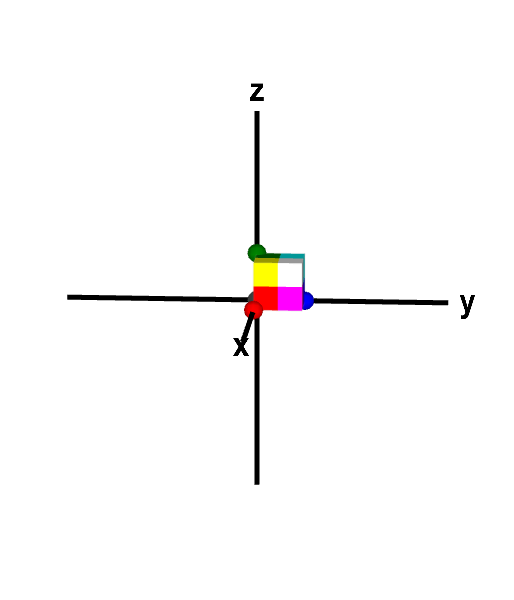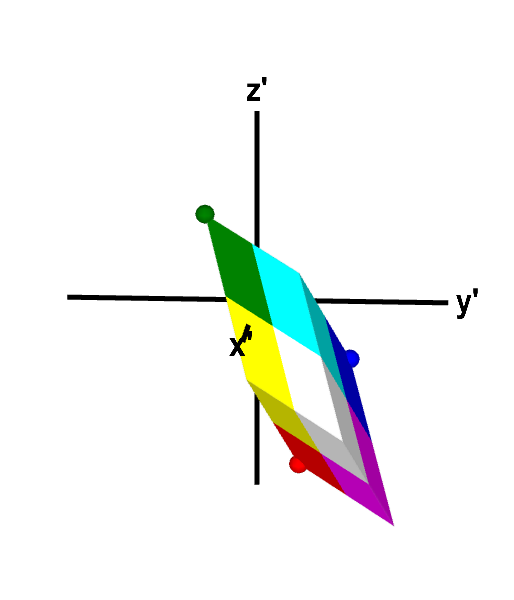# Math Insight

### Applet: A three-dimensional linear transformation that preserves orientationThe linear transformation $\vc{T}(\vc{x}) = A\vc{x}$, where $$A=\left[\begin{array}{rrr}2&1&1\\1&2&-1\\-3&-1&2\end{array}\right]$$ maps the unit cube to a parallelepiped of volume 12. The expansion of volume by $\vc{T}$ is reflected by that fact that $\det A = 12$. Since $\det A$ is positive, $\vc{T}$ preserves orientation, as revealed by the face coloring of the cube and parallelogram. The order of the colors on corresponding faces, when moving in a counterclockwise direction, is the same for both the cube and the parallelepiped. For example, both objects have a face with the counterclockwise color order blue, magenta, white, cyan. You can further explore the mapping by changing either shape to other parallelepipeds by dragging the points on four of its vertices.